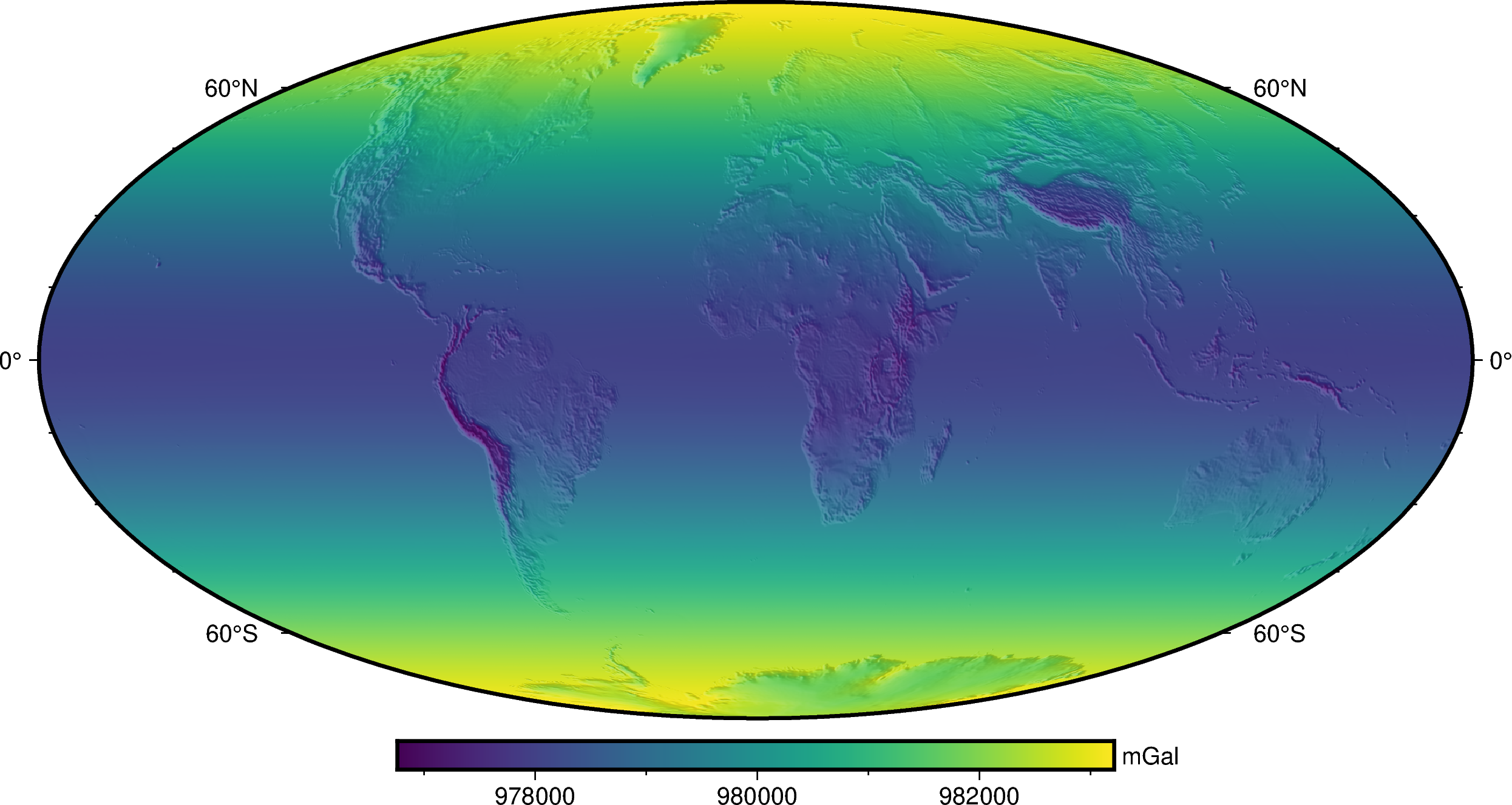# Overview#

## The library#

All functions and classes in Boule are available from the base namespace of the `boule` package. This means that you can access all of them with a single import:

```# Boule is usually imported as bl
import boule as bl
```

## Ellipsoids#

Boule comes with several built-in ellipsoids that are available as global variables in the `boule` module. The ellipsoids can be printed to view their defining attributes:

```print(bl.WGS84)
print(bl.MOON)
print(bl.VESTA)
```
```Ellipsoid(name='WGS84', semimajor_axis=6378137, flattening=0.0033528106647474805, geocentric_grav_const=398600441800000.0, angular_velocity=7.292115e-05, long_name='World Geodetic System 1984', reference='Hofmann-Wellenhof, B., & Moritz, H. (2006). Physical Geodesy (2nd, corr. ed. 2006 edition ed.). Wien\u202f; New York: Springer.')
Sphere(name='MOON', radius=1737151, geocentric_grav_const=4902800070000.0, angular_velocity=2.6617073e-06, long_name='Moon Spheroid', reference='Wieczorek, MA (2015). 10.05 - Gravity and Topography of the Terrestrial Planets, Treatise of Geophysics (Second Edition); Elsevier. doi:10.1016/B978-0-444-53802-4.00169-X')
TriaxialEllipsoid(name='VESTA', semimajor_axis=286300, semimedium_axis=278600, semiminor_axis=223200, geocentric_grav_const=17290940000.0, angular_velocity=0.00032671050958367, long_name='Vesta Triaxial Ellipsoid', reference='Russell, C. T., Raymond, C. A., Coradini, A., McSween, H. Y., Zuber, M. T., Nathues, A., et al. (2012). Dawn at Vesta: Testing the Protoplanetary Paradigm. Science. doi:10.1126/science.1219381')
```

Ellipsoids define a name (short and long version) and reference for the origin of the numbers used:

```print(f"{bl.MARS.name}: {bl.MARS.long_name}")
print(bl.MARS.reference)
```
```MARS: Mars Ellipsoid
Ardalan, A. A., Karimi, R., & Grafarend, E. W. (2009). A New Reference Equipotential Surface, and Reference Ellipsoid for the Planet Mars. Earth, Moon, and Planets, 106(1), 1. doi:10.1007/s11038-009-9342-7
```

Other derived properties of ellipsoids are calculated on demand when accessed:

```print(bl.GRS80.first_eccentricity)
print(bl.GRS80.gravity_pole)
print(bl.GRS80.gravity_equator)
```
```0.08181919104281579
9.832186368517242
9.78032677153605
```

Hint

You may have noticed that there are 3 different types of ellipsoids: `Ellipsoid`, `Sphere`, and `TriaxialEllipsoid`. They each offer different attributes and capabilities. Be sure to check out the List of functions and classes (API) for a full list of what each class offers.

## Normal gravity#

Ellipsoids can be used for computations generally encountered in geodetic and geophysical applications. A common one is calculating normal gravity. Here is an example of using Boule to calculate the normal gravity of the WGS84 ellipsoid on the Earth’s surface (topography in the continents, the geoid the oceans).

First, we need to import a few other packages:

```import ensaio        # For downloading sample data
import pygmt         # For plotting maps
import xarray as xr  # For manipulating grids
```

Now we can download and open co-located topography and geoid grids using `ensaio` and `xarray`:

```fname_topo = ensaio.fetch_earth_topography(version=1)
fname_geoid = ensaio.fetch_earth_geoid(version=1)
geoid
```
```<xarray.DataArray 'geoid' (latitude: 1081, longitude: 2161)>
array([[-29.5     , -29.5     , -29.5     , ..., -29.5     , -29.5     ,
-29.5     ],
[-29.5     , -29.5     , -29.5     , ..., -29.5     , -29.5     ,
-29.5     ],
[-29.6     , -29.6     , -29.6     , ..., -29.6     , -29.6     ,
-29.6     ],
...,
[ 14.7     ,  14.7     ,  14.7     , ...,  14.7     ,  14.7     ,
14.7     ],
[ 15.2     ,  15.2     ,  15.2     , ...,  15.2     ,  15.2     ,
15.2     ],
[ 15.400001,  15.400001,  15.400001, ...,  15.400001,  15.400001,
15.400001]], dtype=float32)
Coordinates:
* longitude  (longitude) float64 -180.0 -179.8 -179.7 ... 179.7 179.8 180.0
* latitude   (latitude) float64 -90.0 -89.83 -89.67 -89.5 ... 89.67 89.83 90.0
Attributes:
Conventions:     CF-1.8
title:           Geoid height (EIGEN-6C4) with respect to WGS84
crs:             WGS84
source:          Generated from the EIGEN-6C4 model by the ICGEM Calculat...
references:      https://doi.org/10.5880/icgem.2015.1
long_name:       geoid height
standard_name:   geoid_height_above_reference_ellipsoid
description:     height of the geoid with respect to the WGS84 ellipsoid
units:           m
actual_range:    [-106.5   86. ]

The computation height can be defined by combining topography and geoid:

```height = xr.where(
topography >= 0,
topography + geoid,  # geometric height of topography in the continents
geoid,  # geoid height in the oceans
)
```

Finally, we can calculate normal gravity using `normal_gravity` at the given heights and plot it on a map with `pygmt`:

```gamma = bl.WGS84.normal_gravity(topography.latitude, height)

fig = pygmt.Figure()